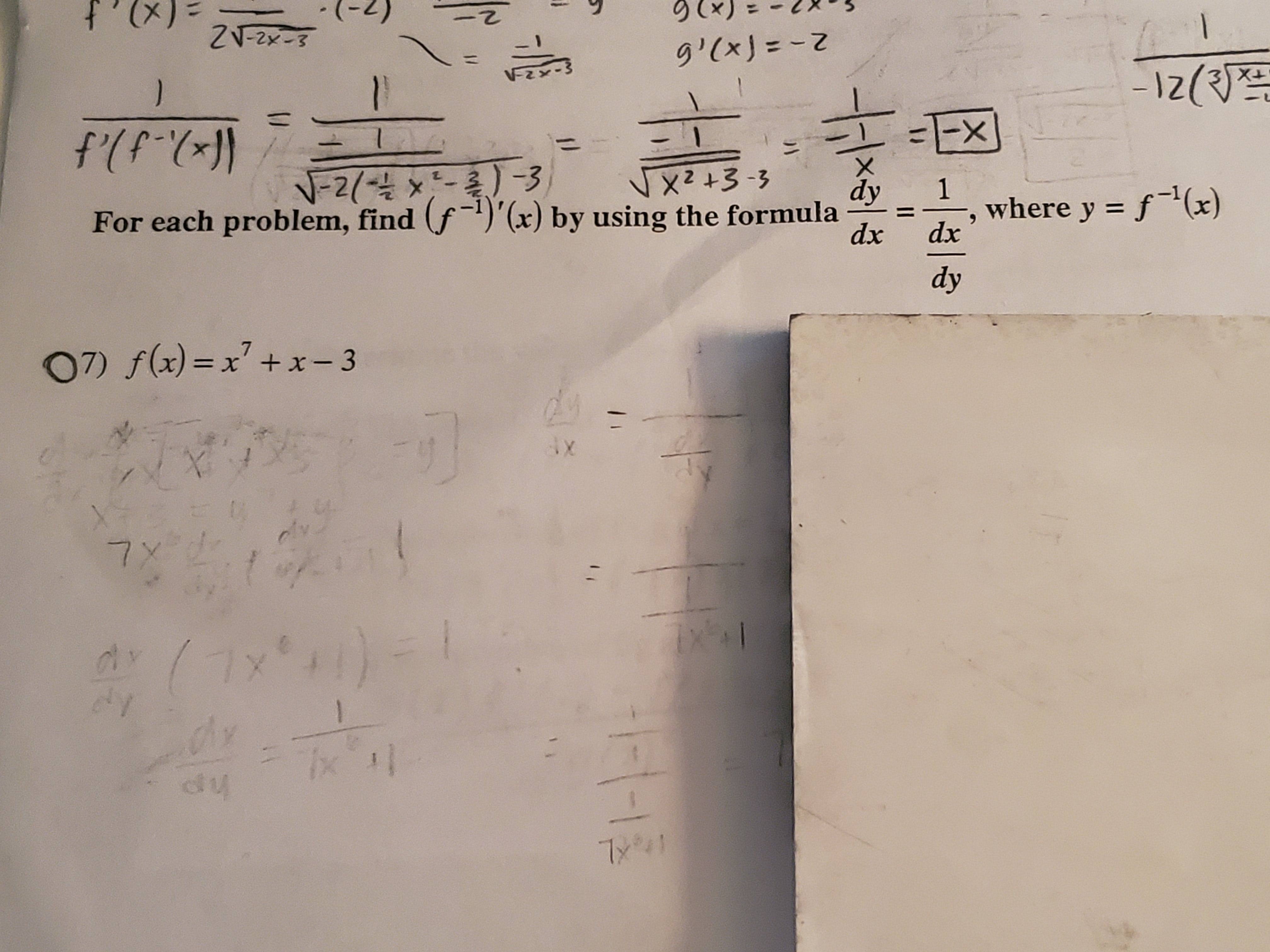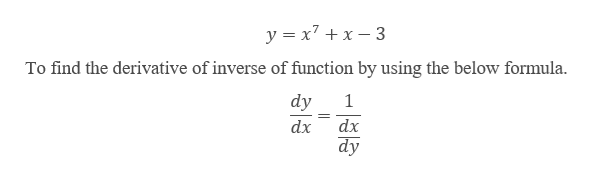# ZV-2x-8g'(xJ=Z)-1z(f(PEXN-2 32- Et zXFor each problem, find (f)'(x) by using the formulady1where y f(x)dxdxdy07) f(x)= x + x-3axdly7 X(ל'אר) -dy7x1/U

Question
54 viewshelp_outlineImage TranscriptioncloseZV-2x-8 g'(xJ=Z ) -1z( f(P EX N-2 3 2- Et zX For each problem, find (f)'(x) by using the formula dy 1 where y f(x) dx dx dy 07) f(x)= x + x-3 ax dly 7 X (ל'אר) - dy 7x 1/ U fullscreen
check_circle

Step 1

Given function:help_outlineImage Transcriptioncloseyx7 3 To find the derivative of inverse of function by using the below formula dy 1 dx dx dy fullscreen
Step 2

Now, replace x by y in th...

### Want to see the full answer?

See Solution

#### Want to see this answer and more?

Solutions are written by subject experts who are available 24/7. Questions are typically answered within 1 hour.*

See Solution
*Response times may vary by subject and question.
Tagged in

### Derivative